## The Rank of a Matrix

The maximum number of linearly independent rows in a matrix A is called the row rank of A, and the maximum number of linarly independent columns in A is called the column rank of A. If A is an m by n matrix, that is, if A has m rows and n columns, then it is obvious thatWhat is not so obvious, however, is that for any matrix A,

the row rank of A = the column rank of A

Because of this fact, there is no reason to distinguish between row rank and column rank; the common value is simply called the rank of the matrix. Therefore, if A is m x n, it follows from the inequalities in (*) that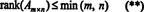where min( m, n) denotes the smaller of the two numbers m and n (or their common value if m = n). For example, the rank of a 3 x 5 matrix can be no more than 3, and the rank of a 4 x 2 matrix can be no more than 2. A 3 x 5 matrix,can be thought of as composed of three 5‐vectors (the rows) or five 3‐vectors (the columns). Although three 5‐vectors could be linearly independent, it is not possible to have five 3‐vectors that are independent. Any collection of more than three 3‐vectors is automatically dependent. Thus, the column rank—and therefore the rank—of such a matrix can be no greater than 3. So, if A is a 3 x 5 matrix, this argument shows thatin accord with (**).

The process by which the rank of a matrix is determined can be illustrated by the following example. Suppose A is the 4 x 4 matrix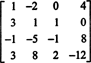The four row vectors,are not independent, since, for example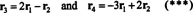The fact that the vectors r 3 and r 4 can be written as linear combinations of the other two ( r 1 and r 2, which are independent) means that the maximum number of independent rows is 2. Thus, the row rank—and therefore the rank—of this matrix is 2.

The equations in (***) can be rewritten as follows:The first equation here implies that if −2 times that first row is added to the third and then the second row is added to the (new) third row, the third row will be become 0, a row of zeros. The second equation above says that similar operations performed on the fourth row can produce a row of zeros there also. If after these operations are completed, −3 times the first row is then added to the second row (to clear out all entires below the entry a 11 = 1 in the first column), these elementary row operations reduce the original matrix A to the echelon form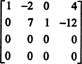The fact that there are exactly 2 nonzero rows in the reduced form of the matrix indicates that the maximum number of linearly independent rows is 2; hence, rank A = 2, in agreement with the conclusion above. In general, then, to compute the rank of a matrix, perform elementary row operations until the matrix is left in echelon form; the number of nonzero rows remaining in the reduced matrix is the rank. [Note: Since column rank = row rank, only two of the four columns in Ac 1, c 2, c 3, and c 4—are linearly independent. Show that this is indeed the case by verifying the relations(and checking that c 1 and c 3 are independent). The reduced form of A makes these relations especially easy to see.]

Example 1: Find the rank of the matrixFirst, because the matrix is 4 x 3, its rank can be no greater than 3. Therefore, at least one of the four rows will become a row of zeros. Perform the following row operations:Since there are 3 nonzero rows remaining in this echelon form of B,Example 2: Determine the rank of the 4 by 4 checkerboard matrix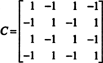Since r 2 = r 4 = −r 1 and r 3 = r 1, all rows but the first vanish upon row‐reduction:Since only 1 nonzero row remains, rank C = 1.